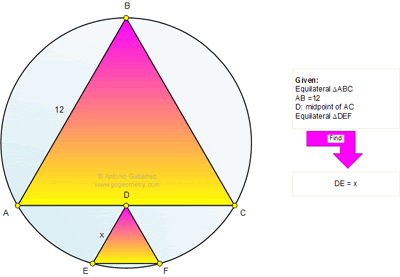## Monday, December 5, 2011

### Problem 700: Equilateral Triangle, Circle, Circular Segment, Midpoint, Metric Relations

Geometry Problem
Level: Mathematics Education, High School, Honors Geometry, College.

Click the figure below to see the complete problem 700.1.http://img37.imageshack.us/img37/9728/problem700.png

FD cut AB and AO at G and H ( see picture)
OA= AG*2/√3 =12/√3
In triangle OHF , applying Pythagoras Theorem we get HF=3. √5
So x=HF-HD=3(√5-1)
Peter Tran

2.Extend ED to cut AC at X and circle at Y
EDXY ∥ AB
So DE = 6 ; and XY = x (by symmetry)